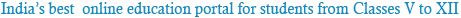### Sample Test Mathematics

1
The Set of all letter in the word “ MATHEMATICS” is :
• 1 {M,A,T,H,E,I,C,S}.
• 2 {M,A,T,H,E,M,A,T,I,C,S}
• 3 None of these
• 4 All of these
2
The set contain { x: x ɛ N and 2 < x < 3} is:
• 1 1
• 2 0
• 3 ɸ
• 4 2.1 to 2.9
3
The set contain { x: x ɛ N and x2 – 9 = 0} is:
• 1 Empty set
• 2 Infinite set
• 3 Singleton set
• 4 it is not any set
4
Represent set D = {2,4,8,16,32} in set builder form :
• 1 D = { x: x ɛ N and 1 < x < 33}
• 2 D = { x: x ɛ Z and 1 < x < 33}
• 3 D = { x: x = 2n , n ɛ N and 0 < n < 6}
• 4 D = { x: x = 2n , n ɛ Z and 0 < x < 6}
5
Which of the following collection are set :
I - Collection of 11 best player of Indian team

II - The collection of all rich woman in the world
• 1 Only I
• 2 Only II
• 3 Both I & II
• 4 None of these
6
Which of the following collection are set :
I - Collection of all the days of the week staring with “T”

II - The collection of all player who score more than 23 centuries in International cricket match
• 1 Only I
• 2 Only II
• 3 Both I & II
• 4 None of these
7
Choose the correct equivalence of n(A ∪ B ∪ C) :
• 1 {n(A) + n(B) + n(C) + n(A ∩B ∩C)} – {n(A ∪ B) + n(B ∪C) + n(A ∪C) }
• 2 {n(A) + n(B) + n(C) + n(A ∩B ∩C)} – {n(A ∩ B) + n(B ∩C) + n(A ∩C) }
• 3 {n(A) + n(B) + n(C) + n(A ∪B ∪C)} – {n(A ∩ B) + n(B ∩C) + n(A ∩C) }
• 4 {n(A) + n(B) + n(C) + n(A ∪B ∪C)} – {n(A ∪ B) + n(B ∪C) + n(A ∪C) }
8
Choose the correct equivalence of n(A∪B), A∩B = ɸ :
• 1 n(A) + n(B)
• 2 n(A) - n(B)
• 3 n(A) ∩ n(B)
• 4 n(A) ∪ n(B)
9
Which of the following collection are set is valid if A ⊂ U:
I : U’ = ɸ M
II : A∪A’ = U
• 1 Only I
• 2 Only II
• 3 Both I & II
• 4 None of these
10
A ∪ (B∩C) = (A∪B) ∩ (A∪C) is :
• 1 Union distributive intersection
• 2 Union distributive intersection
• 3 Union associative intersection
• 4 Union associative intersection

Powered by: iVerticals Web Technology Pvt Ltd